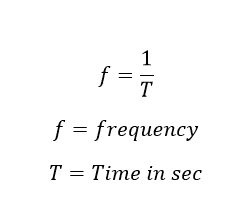# What is Frequency:

The number of cycles completed per second by an alternating quantity is known as frequency and is denoted by f. In SI system, the frequency is expressed in Hz that is the short form of hertz (pronounced as hurts). The wave’s frequency is said to be One hertz (or Hz), which is equal to one cycle per second. The number of cycles completed per secondAlso, the reciprocal of the time period can be called as frequency.

The general utility of AC power supply system is produced at a frequency of 50 Hz or 60 Hz. But in the world, the major country uses 50 Hz power system, In US, Brazil, Canada such countries use 60 Hz power system.

Also, see: 50 Hz power system vs 60 Hz power system. Why India has 50 Hz power system.

The output of the equipment increases with the increase in frequency. The output voltage is directly proportional to the frequency of the machine. For a given output, smaller size machines are required as compared to those for lower frequency output. Because of high power-weight ratio, the relative cost of the equipment is also reduced.

Learn More:   Motor Hp (Horse Power) Calculator DC, Single Phase & Three phase

At the same time increase in frequency, the loss of the electrical circuit also increases. But the size of the machine decreases

Example: HF Transformer is used in SMPS circuit

Lower regulation, lower skin effect resulting in lower ohmic losses (resistive losses), lower magnetic and dielectric losses resulting in higher efficiency, lower corona loss and higher power transmission line capacity as compared to those at the higher frequency.

## Time Period versus frequency

As a matter of convenience, longer and slower waves, such as ocean surface waves, tend to be described by wave period rather than frequency. Short and fast waves, like audio and radio, are usually described by their frequency instead of the period. These commonly used conversions are listed below:

 Frequency 1 mHz (10−3 Hz) 1 Hz (10 Hz) 1 kHz (103 Hz) 1 MHz (106 Hz) 1 GHz (109 Hz) 1 THz (1012 Hz) Period 1 ks (103 s) 1 s (10 s) 1 ms (10−3 s) 1 µs (10−6 s) 1 ns (10−9 s) 1 ps (10−12 s)

## Frequency measurement:

Frequency can be measured by following ways,

Counting:

Calculating the frequency of a repeating event is accomplished by counting the number of times that event occurs within a specific time period, then dividing the count by the length of the time period. For example, if 71 events occur within 15 seconds the frequency is:

Stroboscope:

An older method of measuring the frequency of rotating or vibrating objects is to use a stroboscope. This is an intense repetitively flashing light (strobe light) whose frequency can be adjusted with a calibrated timing circuit.

Frequency counter:

This is the most used method to measure the frequency of the circuit. Higher frequencies are usually measured with a frequency counter. This is an electronic instrument which measures the frequency of an applied repetitive electronic signal and displays the result in hertz on a digital display.

Heterodyne methods:

Above the range of frequency counters, frequencies of electromagnetic signals are often measured indirectly by means of heterodyning (frequency conversion).